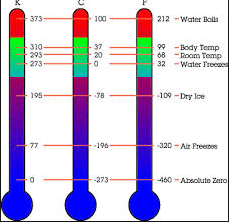Thermodynamics

# 3 types of temperature scalesTemperature is the degree of hotness and coldness of an object. The SI unit of temperature is Kelvin. There are three types of temperature scales, Celcius temperature scales, Fahrenheit temperature scales, and kelvin temperature scales.

## Temperature Scales List

Here’s the list of all types of temperature scales

• Celcius temperature scales
• Fahrenheit Temperature scales
• Kelvin Temperature Scales

Temperature is one of the seven base units, and we might, therefore, attempt to treat temperature as we treated other base units in the S.I system; establish a standard and relate all other scales to the standard. However, the temperature has a nature different from that of other S.I base units, and so this scheme will not work in quite that simple a form.

For instance, if we define one period of vibration of the light emitted by a Cesium atom as a standard of time, then two such vibrations last for twice the time, and any arbitrary time interval can in effect be measured in terms of the number of vibrations. But even if we define a standard of temperature, such as that of water boiling under certain conditions we have no procedure to determine a temperature twice as large.

Two pots of boiling water, after all, have the same temperature as one pot. There is no apparent way using only this standard that we can relate the temperature of boiling water to that, for example, of boiling oil; no amount of boiling water will ever be in thermal equilibrium with boiling oil.

To establish a measuring scale for temperatures we adopt the following procedure, which differs from the usual procedure for the S.I base units; we find a substance that has a property that varies temperature, and we measure that property. The substance we choose is called the thermometric substance, and the property that depends on temperature is called thermometric property.

## What are the three types of temperature scales?

A scale is marked on the thermometer. The temperature of the body in contact with the thermometer can be read on that scale. Three scales of temperature are in common use. These are:

• Celsius scale or centigrade scale
• Fahrenheit scale
• Kelvin scale
1. Watch video to see temperature scales:

• ### Celsius scale or degree centigrade

In nearly all countries of the world, the Celsius scale or degree centigrade is used for all popular and commercial and most scientific measurements. Anders Celsius (1701-1744) was a Swedish astronomer who, in addition to developing the temperature scale named for him, made measurements of the length of the arc of a median that verified Newton’s theory of the flattering of the earth at the poles. On the Celsius scale, the interval between lower and upper fixed points is divided into 100 equal parts. The lower fixed point is marked as O °C and the upper fixed point is marked as 100 °C. It means on the Celsius scale the freezing point of water is O °C and the boiling point of water is 100 °C.

• ### The Fahrenheit scale

The Fahrenheit scale,  used in the United States, employs a smaller degree than the Celsius scale, and its zero is set to a different temperature. Daniel Fahrenheit (1686-1744) , a contemporary of Celsius, was a German physicist who invented both the alcohol and mercury liquid thermometers and used them to study the boiling and freezing points of liquids. On this scale, the freezing point of water is 32 degrees and the boiling point of water is 212 degrees. The Fahrenheit scale is divided into 180 equal parts.

• ### Kelvin scale

in SI units, the unit of temperature is Kelvin(k) and its scale is called the kelvin scale of temperature. the interval between the lower and upper fixed points is divided into 100 equal parts. Thus, a change in 1°c is equal to a charge of 1 K. The lower fixed point on this scale corresponds to 273 Kn and the upper fixed point is referred o 373 K. The zero on this scale is called the absolute zero and is equal to _273°C. At the absolute 0, all thermometric properties are zero. There would be no kinetic energy.